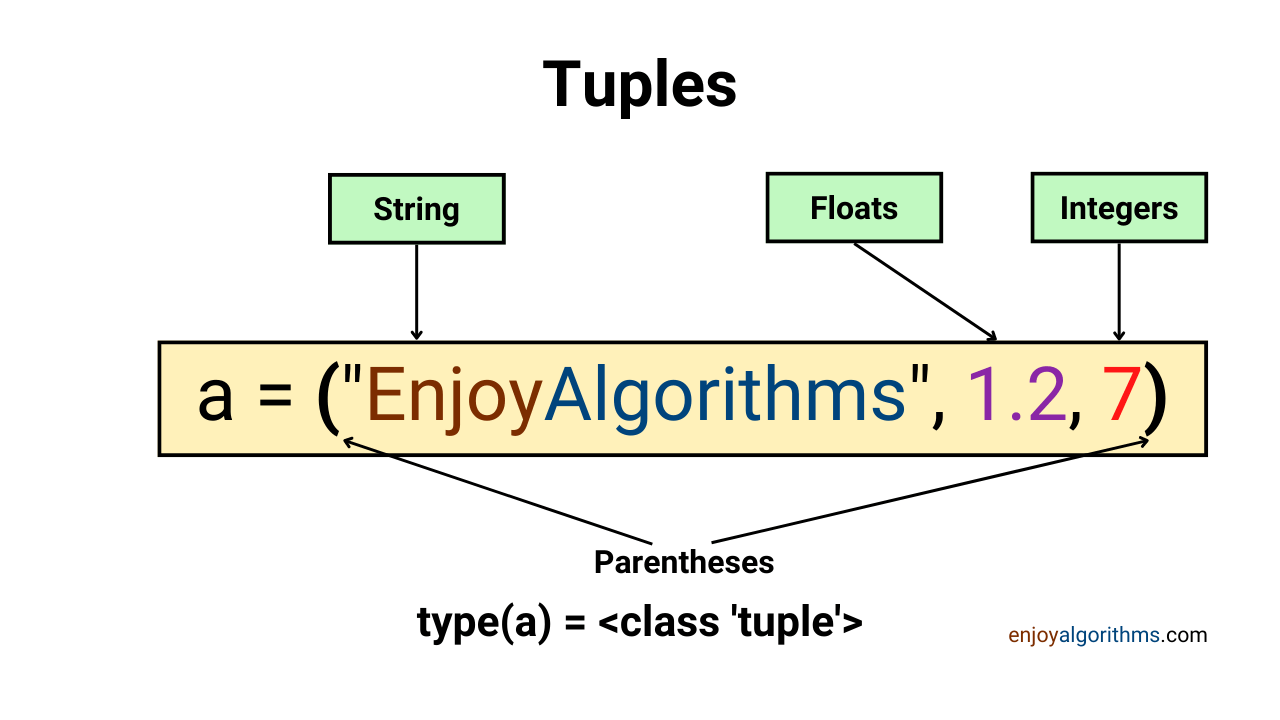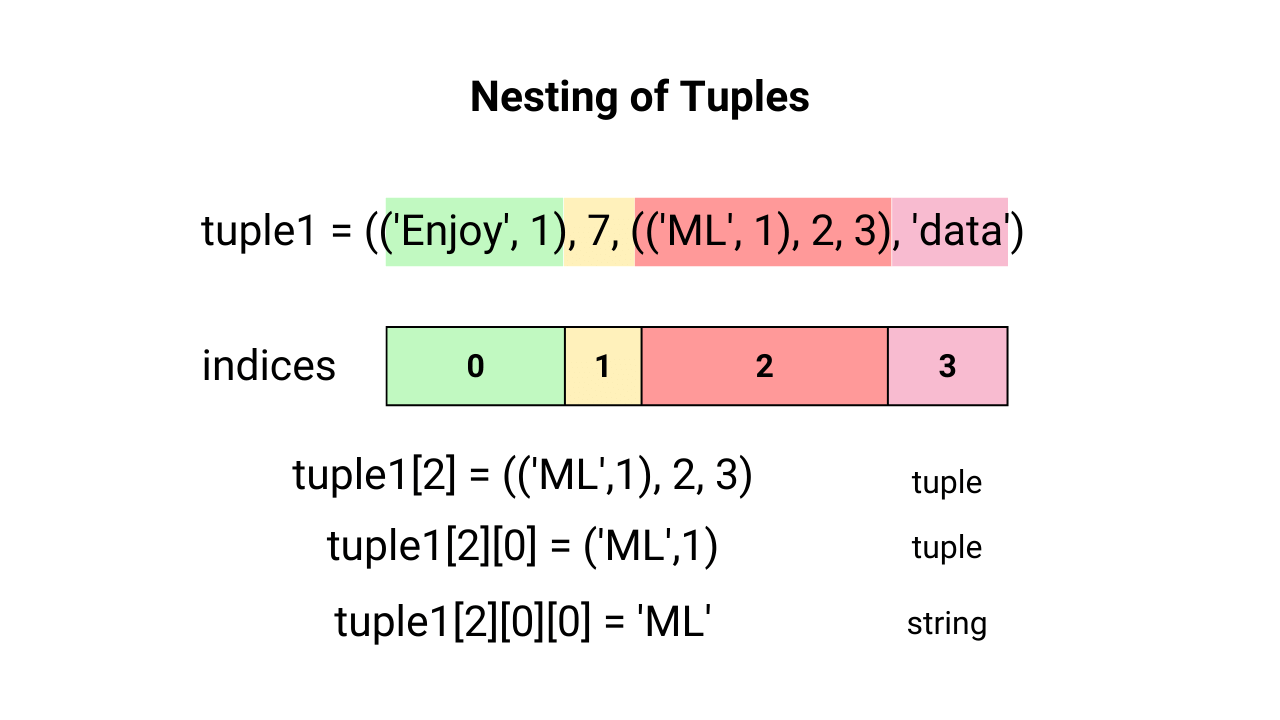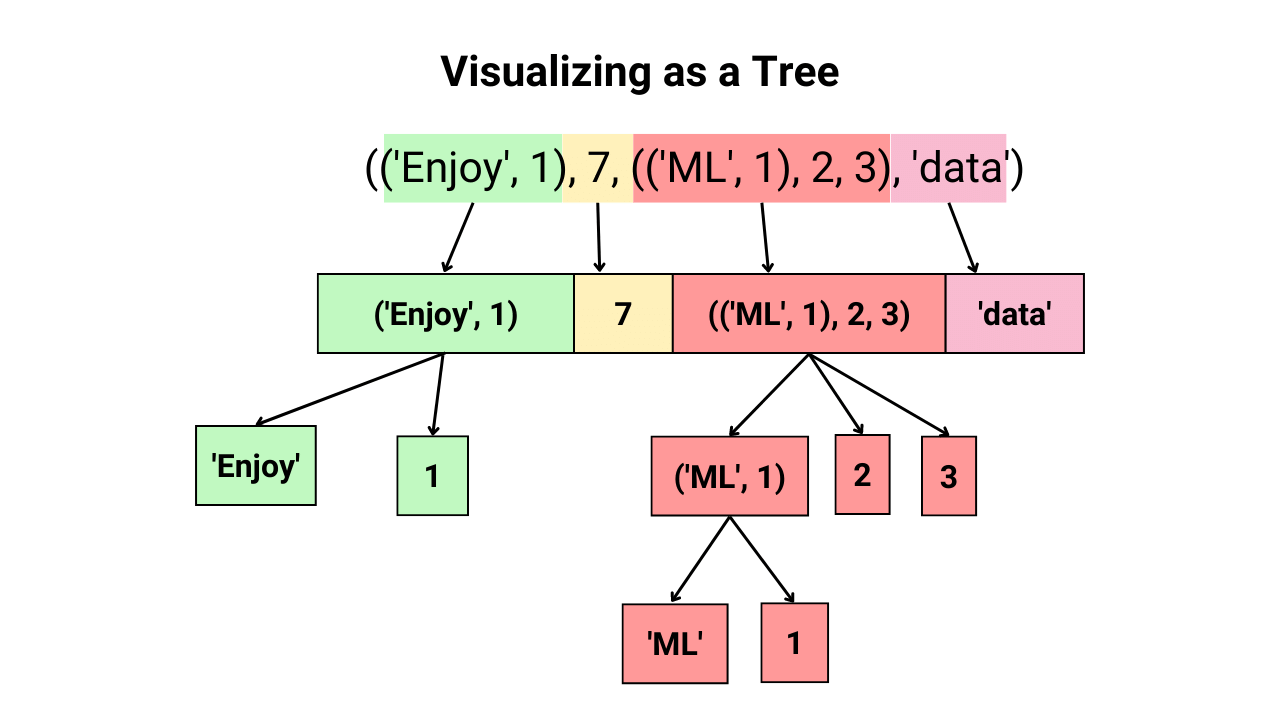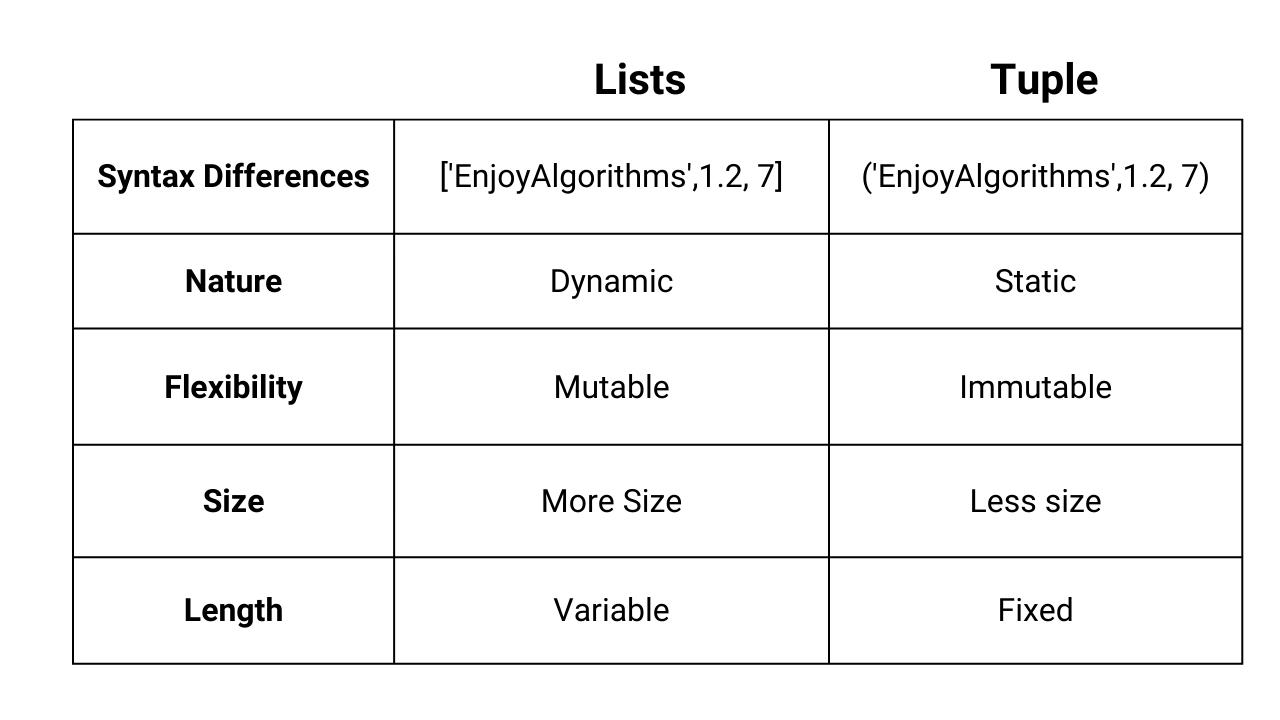# Python Data Structures: Tuples and Lists

We use various data types like Numbers (Integer & Float), Boolean, and Strings to write programs in any language. But one question should be coming into our mind, how to store these data types in our computers?

Variables would be an option, like assigning the value corresponding to a particular data type to a variable and storing it. But there are more efficient ways to store a larger number of data types. For example, if we store 100 float values, we will have to make 100 variables and remember them for later use, which is highly cumbersome. Hence we need the help of data structures which is an efficient way of organizing data in our computers.

In this article, we will discuss two data structures (tuples and lists) in Python and their use in Machine Learning and Data Science domains. These data structures are also called compound data types because they can store all primitive data types like Strings, ints, and floats.

### Key takeaways from the blog

After going through this blog, we will be able to understand the following things:

1. What are tuples?
2. What are mutable and immutable data structures?
3. What are the various operations performed on tuples?
4. What are lists?
5. What are the various operations performed on lists and tuples?

### Tuples

Tuples are an ordered sequence of the same or mixed data types enclosed in the smaller parenthesis, "( )". An example of a tuple is shown in the image below, which contains 3 different data type elements.#### Defining Tuples in Python

We need to enclose the comma-separated values inside the small parenthesis. For example,

``````>>> a = ('EnjoyAlgorithms', 1.2, 7)
>>> type(a)
<class 'tuple'>

# We can also define an empty tuple like this
>>> a = ()
>>> type(a)
<class 'tuple'>``````

And as they can contain various primitive data types, they form a compound data type named 'tuple'.

During the definition of tuples, there are certain things that we should take care of. When we try to define a tuple with a single item, it will store it as its native data type, not as a tuple. Still, if we want to store it as a tuple only, we need to place an additional ",". For example:

``````>>> a = (7)
>>> type(a)
<class 'int'>

>>> a = ('EnjoyAlgorithms')
>>> type(a)
<class 'str'>

>>> a = ('EnjoyAlgorithms',)
>>> type(a)
<class 'tuple'>

>>> a = (7,)
>>> type(a)
<class 'tuple'>

## Also, if we don't want to place parenthesis,
## then python will store values as tuples only
>>> a = 1, 2, 3
>>> type(a)
<class 'tuple'>``````

Depending on the limit of our computer's memory, a tuple can contain any number of elements. Tuple is a form of data structure, and we constantly need to access its contents or the data it stores. But before that, let's first understand what an index is in Tuples and Lists.

#### Index in Python Tuples and Lists

We store multiple values using list and tuple data structures in Python. We use the indices to state the position or location of that stored value. In Python, index values start from 0 until the length of tuple -1. For example, in the image above, we had a tuple containing three values ("EnjoyAlgorithms", 1.2, 7), where 'EnjoyAlgorithms' is at the 0th index, 1.2 is at the 1st index, and 7 is at the 2nd index.

Now let's see how these index values will help in extracting the elements from tuples.

#### Access elements from a Defined Tuple

To access the elements in the tuple, we can use the name of the tuple followed by the square bracket and the index number for the element we want to access. For example, if there is a variable tuple1 = ("EnjoyAlgorithms", 1.2, 7), we can access the first element as tuple1, which will give the output as "EnjoyAlgorithms". Similarly, to access the second and third elements, tuple1 and tuple1 can be used, respectively.

Please note that to access the last element, we use the index as the length of tuple -1. This is the maximum index we can use to extract the number from a tuple. We can use the "len" function to get the length of the tuple. From the earlier example, if we try to use tuple1, it will produce an IndexError.

``````>>> tuple1 = ('EnjoyAlgorithms', 1.2, 7)
>>> tuple1
7
>>> len(tuple1)
3

>>> tuple1
Traceback (most recent call last):
File "<stdin>", line 1, in <module>
IndexError: tuple index out of range``````

#### Negative indices in Python

We can also use negative indices, representing that we are reading the tuple starting from the last element in reverse order. Let's consider the same example of tuple1. The last element can also be accessed by tuple1[-1]. The minimum value of the index via this method can be (-length of tuple), which is -3 in our case, tuple1 = tuple1[-3].

#### Accessing Elements after fixed intervals in Python

There is one more advanced way of extraction. What if someone tells us to extract every element at the odd indices? Please note that the indices in python start from 0, so odd indices mean 1, 3, 5, 7, and so on. We can use a double colon to access elements in this order. If "a" is a tuple, and we want to access every Kth element starting from the ith element, we can write it in a more generalized form [i::K]. These types of accesses are used in Data Science when we try to reduce the number of data instances to form the final set.

``````>>> a = (0, 3, 5, 7, 9, 8, 10, 11, 12, 15, 7)
>>> a[1::2]
(3, 7, 8, 11, 15)``````

#### Reversing the Tuple

We can easily reverse any given tuple using the same double colon technique. We need to traverse the tuple from the last using negative indices. This technique is beneficial and can be found in many ML and Data Science codes. For example, we pass the data in reversed order when we want to predict the unknown past using Machine Learning.

``````>>> a = (0, 3, 5, 7, 9, 8, 10, 11, 12, 15, 7)
>>> a[::-1]
(7, 15, 12, 11, 10, 8, 9, 7, 5, 3, 0)``````

#### Slicing a Tuple

It can be a possibility that the length of the tuple is high, and we need values present between two indices. In simple terms, we are extracting the range of indices from a tuple. To do that, we use the method of slicing. For example, in the code below, tuple1 is our tuple, and if we want to extract the elements in the range of the 2nd to the 4th index, then we need to pass the command as tuple1[2:5].

Please note that we are using 2:5 to extract the elements until the 4th index, not 2:4. In Python, we start counting from 0, not from 1. If we want to access elements starting from the 0th index, we have two options, tuple1[0:5] or tuple1[:5]. Similarly, if we don't mention the second integer, it will give the slice till the last index, tuple1[2:].

``````>>> tuple1 = ('EnjoyAlgotihms', 1.2, 7, 'ML', 11, 'Data Science', 1.1)

>>> tuple1[2:5]
(7, 'ML', 11)

>>> tuple1[:5]
('EnjoyAlgotihms', 1.2, 7, 'ML', 11)

>>> tuple1[2:]
(7, 'ML', 11, 'Data Science', 1.1)``````

Slicing is very helpful in Data Science, especially when we want to select a particular data window from the available dataset.

#### Addition or Concatenation of Tuples

Concatenation means placing the data of two tuples inside a single tuple. For that, let's take an example,

``````>>> tuple1 = ('EnjoyAlgorithms', 1.2, 7)
>>> tuple2 = ('Machine', 'learning', 101)

>>> concatenated_tuple = tuple1 + tuple2
>>> concatenated_tuple
('EnjoyAlgorithms', 1.2, 7, 'Machine', 'learning', 101)``````

In Machine Learning and Data Science, data is stored in multiple files. When we store different datasets in multiple tuples and want our Machine to be trained on all these datasets, then we use the concatenation of tuples.

#### Objects in Python

As we discussed that everything in Python is an object which contains three basic properties,

• Identity: Computer memory location where that object is stored.
• Type: This is the data type that gets attached when we create an object, like string, int, float, etc.
• Value: This is the value stored in the object. For example, in a = 1, the variable a has a value of 1.

Tuples are not an exception here; they are also objects with a 'tuple' data type.

#### Mutable vs Immutable Objects in Python

Identity and Type are two properties attached to an object since its creation. The only thing that can be changed later is its value. If the data type allows us to change the value, it is mutable; if not, then that data type is immutable. Immutable data type examples are integers, float, strings, and tuples. Mutable data types are lists, sets, and dictionaries, which we will see in our consecutive blogs.

As we said, tuples are immutable, which means we can not change the value of any tuple. For example, if we try to change the first element of any tuple by assigning the updated value to the first element, it will throw a TypeError.

``````>>> tuple1 = ('EnjoyAlgorithms', 1.2, 7)

>>> tuple1 = 'ML'
Traceback (most recent call last):
File "<stdin>", line 1, in <module>
TypeError: 'tuple' object does not support item assignment``````

But we must be thinking, how to change the values then? It's an important operation! Our only option is to create a new tuple with the updated values. For example,

``````tuple1 = ('EnjoyAlgorithms', 1.2, 7)

# if we want to change the value inside tuple, we would need to
# create a new tuple.
# We want to change the first value to 'ML'
tuple2 = ('ML', 1.2, 7)``````

#### Nesting of Tuples

One of the exciting things about compound data types is that they can store multiple tuples inside a tuple. If we want to access the elements in the tuple stored in another tuple, we need to place the indices in subsequent square brackets. An example is shown in the image below.We can also visualize the nesting operation as a tree, and after splitting, nodes will look like this:#### Tuples are static

We can not change the values inside tuples because of their immutable nature. If we need to change the size or accommodate more values, we need to make another tuple. Hence, tuples are static.

Now let's see another data structure, 'Lists', which are mutable and the most used compound data type in ML and Data Science.

### Python Lists

Lists are another data structure in Python that stores an ordered sequence of similar or different data type python objects. If we are familiar with arrays in computer science, lists are similar to them but have a more flexible nature. It is Python's most used data structure, so let's understand it in more detail.

#### Defining Lists

We need to enclose the comma-separated values inside the square parenthesis. For example,

``````>>> a = ['EnjoyAlgorithms', 1.2, 7]
>>> type(a)
<class 'list'>

# We can also define an empty lists like this
>>> a = []
>>> type(a)
<class 'list'>``````

Per our computer's memory limit, a list can contain any number of python objects. Many properties and operations that can be performed on lists are similar to those we served in tuples. Still, let's see the variations through examples.

#### Accessing elements from a list

It follows the same rule as tuples. We can give positive and negative indices, which will output the corresponding element.

``````>>> list1 = ['EnjoyAlgorithms', 1.2, 7]
>>> list1
7
>>> len(list1)
3

>>> list1
Traceback (most recent call last):
File "<stdin>", line 1, in <module>
IndexError: list index out of range``````

#### Accessing Elements after fixed intervals

As we did in tuples, we need to provide the starting index integer value and the gap value followed by a colon sign.

``````>>> a = [0, 3, 5, 7, 9, 8, 10, 11, 12, 15, 7]
>>> a[1::2]
[3, 7, 8, 11, 15]``````

#### Reversing the List

``````>>> a = [0, 3, 5, 7, 9, 8, 10, 11, 12, 15, 7]
>>> a[::-1]
[7, 15, 12, 11, 10, 8, 9, 7, 5, 3, 0]``````

#### Slicing of lists

``````>>> list1 = ['EnjoyAlgotihms', 1.2, 7, 'ML', 11, 'Data Science', 1.1]
>>> list1[2:5]
[7, 'ML', 11]

>>> list1[:5]
['EnjoyAlgotihms', 1.2, 7, 'ML', 11]

>>> list1[2:]
[7, 'ML', 11, 'Data Science', 1.1]``````

#### Addition or Concatenation of Lists

``````>>> list1 = ['EnjoyAlgorithms', 1.2, 7]
>>> list2 = ['Machine', 'learning', 101]

>>> concatenated_list = list1 + list2
>>> concatenated_list
['EnjoyAlgorithms', 1.2, 7, 'Machine', 'learning', 101]``````

#### Lists are mutable

Unlike tuples, we can modify the elements of the lists. This is one of the major differences between lists and tuples, as tuples were immutable. Because of this flexibility, lists are more popular than tuples.

``````>>> list1 = ['EnjoyAlgorithms', 1.2, 7]
>>> list1 = 'ML'
### If we notice, earlier in case of tuple, this was
### giving us TypeError but now in case of lists,
### the values got updated.

>>> list1
['ML', 1.2, 7]``````

Now, as the lists are mutable, we can change values inside the lists either one at a time or multiple values directly using the slicing. For example,

``````>>> a = ['EnjoyAlgorithms', 1.2, 7, 'Machine', 'learning', 101]
>>> a[2:5] = [0, 1, 2]
>>> a
['EnjoyAlgorithms', 1.2, 0, 1, 2, 101]``````

#### Nesting of Lists

Similar to tuples, lists can contain one or more lists inside them and can be accessed via subsequent square brackets. The same "tree" representation can be used to illustrate the nesting of lists. An example of accessing the elements from a nested list is shown below.

``````>>> a = [['Enjoy', 1], 7, [['ML', 1], 2, 3], 'data']
>>> type(a)
<class 'list'>

>>> a
[['ML', 1], 2, 3]

>>> a
['ML', 1]

>>> a
1``````

#### Methods to Modify a list

Several in-built methods in Python can be used to modify the lists. Some popular ones are:

• Append method: We can add single objects at the end of the lists using the append function. For example,
• ``````a = ['EnjoyAlgorithms', 1.2, 7, 'Machine', 'learning', 101]
>>> a.append('Data')
>>> a
['EnjoyAlgorithms', 1.2, 7, 'Machine', 'learning', 101, 'Data']

# These functions do not return a new list,
# instead modify the same list. If we define the new list
# like that shown in the example below, it will be not
# assign the values of a to b.

>>> b = a.append('Science')
>>> b
None

>>> a
['EnjoyAlgorithms', 1.2, 7, 'Machine', 'learning', 101, 'Data', 'Science']``````

Please note that the append method can add a single object to lists. So if we try appending multiple objects, it will treat the complete set of multiple objects as a single object and append that.

Append is frequently used while building ML and Data Science applications. For example, developers prefer list append methods when we need to process each sample and then store the processed data in a data structure.

``````>>> b = ['Data', 'Science']
>>> a.append(b)
>>> a
['EnjoyAlgorithms', 1.2, 7, 'Machine', 'learning', 101, ['Data', 'Science']]``````
• Extend Function: Extend Method: As we discussed, the append method can add single objects; hence, a new method was formed: extend. Using this, we can extend the original list with the new list. For example:

``````>>> a = ['EnjoyAlgorithms', 1.2, 7, 'Machine', 'learning', 101]
>>> b = ['Data', 'Science']
>>> a.extend(b)
>>> a
['EnjoyAlgorithms', 1.2, 7, 'Machine', 'learning', 101, 'Data', 'Science']
# This is same as the "+" operator but changing the list inplace.
# We can say that this works as "+=" operator in codes.``````
• Insert method: We want to make room for a new entry at any given index. But the problem is that the index is already occupied. Here, the insert method becomes handy. For example:

``````>>> a = ['EnjoyAlgorithms', 1.2, 7, 'Machine', 'learning', 101]
>>> a.insert(2, 'Data')
>>> a
['EnjoyAlgorithms', 1.2, 'Data', 7, 'Machine', 'learning', 101]``````

The insert method takes two arguments: the first says the index at which we want to insert, and the second is the value we want to insert.

• Remove method: We have seen many methods to insert the values inside lists, but sometimes we also need to remove elements from the list. The first method is ".remove(object)".

``````>>> a = ['EnjoyAlgorithms', 1.2, 7, 'Machine', 'learning', 101]
>>> a.remove('Machine')
>>> a
['EnjoyAlgorithms', 1.2, 7, 'learning', 101]

# If we place object inside remove function that
# does not exists inside the list, it will throw an error.

>>> a.remove('Data')
Traceback (most recent call last):
File "<stdin>", line 1, in <module>
ValueError: list.remove(x): x not in list``````
• Pop method: Now, we must be thinking about what if we don't know the exact object but know the location from which we want to remove the object. In such cases, the pop method helps.

``````>>> a = ['EnjoyAlgorithms', 1.2, 7, 'Machine', 'learning', 101]
>>> a.pop(-1)
101

>>> a
['EnjoyAlgorithms', 1.2, 7, 'Machine', 'learning']

>>> a.pop(2)
7

>>> a
['EnjoyAlgorithms', 1.2, 'Machine', 'learning']``````

#### Lists are Dynamic

If we followed everything until here, we could sense that the lists are dynamic as they can extend to accommodate more values inside them, and they can shrink if we have fewer data samples as we do not need to make new lists for every smaller change. Hence, lists are dynamic.

### Heterogeneous Nesting of Lists and Tuples

Lists and Tuples can contain other lists or tuples inside them. A list can include one or multiple tuples and vice-versa. For example:

``````>>> a = ['EnjoyAlgorithms', ('Data', 'Structures'), ('Machine', 'Learning')]
>>> type(a)
<class 'list'>

>>> a = ('EnjoyAlgorithms', ('Data', 'Structures'), ['Machine', 'Learning'])
>>> type(a)
<class 'tuple'>``````

#### Memory Size Comparison of lists and tuples to store the same data

Tuples are more memory efficient when compared to lists for storing the same information. Let's compare the memory required to store the same information fairly. We can use the __sizeof__() function supported by both data structures.

``````>>> a = ['EnjoyAlgorithms', 1.2, 7, 'Machine', 'learning', 101]
>>> type(a)
<class 'list'>

>>> b = ('EnjoyAlgorithms', 1.2, 7, 'Machine', 'learning', 101)
>>> type(b)
<class 'tuple'>

>>> print('a=',a.__sizeof__())
a= 88

>>> print('b=',b.__sizeof__())
b= 72``````

### Overall comparison between tuples and lists in Python

• Lists are enclosed by squared parenthesis, while tuples are enclosed by small parenthesis.
• Lists are dynamic in nature, as they can be modified at any point inside our code. On the other hand, tuples are static in nature. If we want to modify the tuple, we need to make another one for that.
• Lists are mutable because we can change the values of lists directly. On the other hand, tuples are immutable, and to change the value of an element inside a tuple data structure, we need to make another tuple with an updated value.
• Lists require more memory to store the same data values than Tuples.
• Lists can be extended in our code, but tuples do not provide such flexibility.### Conclusion

This article discussed the two famous data structures used in Python, especially in Machine Learning and Data Science fields, Lists and Tuples. We learned about the various operations performed on these data types and accessing elements from them. We will discuss two other data structures, sets, and dictionaries in the subsequent series. Till then, Enjoy Learning!

Enjoy learning!

☆ 16-Week Live DSA Course
☆ 10-Week Live DSA Course